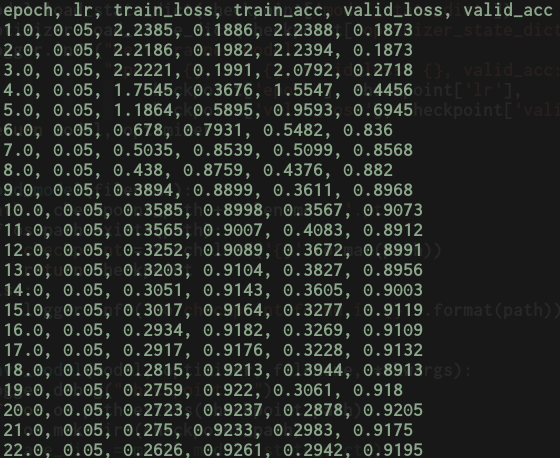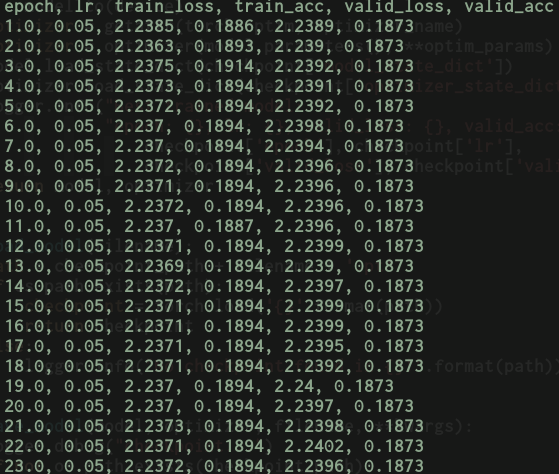# Weird learning stagnation when using DataParallel

I’m currently facing a weird behaviour which I cannot explain. I’m training a vgg16 on svhn and training on 1 gpu with SGD and fixed hypeparams I get the following nice results:Now trying to train the same model with same optimizer and hyperparams as before but using `DataParallel` it exhibits the following behaviour where the learning process actually stagnates and it doesn’t learn anything.Even more weird is the fact that if I swap vgg16 for resnet50 it starts learning again.

Anyone has any insights on this, or what it might be going on?

I would expect that if a model `M` trained on one device with fixed optimizer and hyperams exhibiting good learning behaviour to have the same behaviour when trained with `DataParallel` using same optimizer and hyperparams.

Could you post a minimal working example to reproduce these results? It could be, e.g. that you don’t pass the DataParallel’s parameters to the `params` argument of the optimizer

@dsuess Thanks for the response!
I’ve actually followed the example here for `DataParallel`.

Here’s a MWE (trying to avoid putting lot’s of code here)

``````model = VGG16
dataset = CIFAR10

def main():
model = create_model()
optimizer = torch.optim.SGD(model.parameters(), lr=0.05, weight_decay=5e-4, momentum=0.9)
if torch.cuda.device_count() > 1:
model = torch.nn.parallel.DataParallel(model).to(device)
else:
model.to(device)
train()
test()
``````

I think that might be the case since in the MWE I’m creating the optimizer before putting the model on DataParallel?

But, it doesn’t explain why the same code works when everything else is constant in the MWE and just swap VGG16 for ResNet50?

Let me give it a try and rearrange the optimizer order after model is sent to DataParallel.

Last question, do you by any chance have any insight on this problem?

Thanks!

It looks like a dimension problem to me. Have you check that the batch sizes are at the same position?

What do you mean by at the same position?
My understanding is that `DataParallel` takes data of batch size `m` and splits them as `int(m/num_of_devices)` sending to each device it’s own split and a copy of the model?

OK, just double checked and your order seems to be correct. I think it’s best if you post a full working example, otherwise we’re just guessing

Sorry, I mean have you rule out the possibility of shape mismatch?

Ok so I did check and reordered the code as below:

``````model = VGG16
dataset = CIFAR10

def main():
model = create_model()